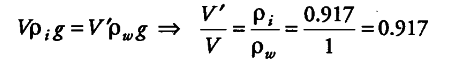# Iceberg floats in water with part of it submerged

Iceberg floats in water with part of it submerged. What is the fraction of the volume of iceberg submerged, if the density of ice is ρ, = 0.917 g \${{cm}^{3}}\$

Given, density of ice (p, ) = 0.917 g/ {{cm}^{3}}
Density of water = 1 g/ {{cm}^{3}}
Let V be the total volume of the iceberg and V’ of its volume be submerged in water.
In floating condition,
Weight of the iceberg = Weight of the water displaced by the submerged part by ice